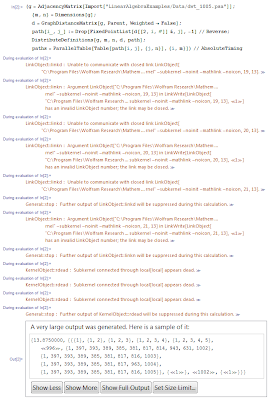## Friday, 30 July 2010

### All pairs shortest paths

The all-pairs shortest paths algorithm from graph theory computes the shortest paths from every vertex in a graph to every other vertex. The graph may be directed or undirected and may have weighted edges and even negative weights. The algorithm used is called Floyd-Warshall and it takes O(n3) time and O(n2) space where n is the number of vertices in the graph.

Although Wolfram Research cheekily worked this example into the Numb3rs TV show, Mathematica does not actually provide a complete implementation of the algorithm required to obtain the shortest paths but, rather, is only able to return the path lengths and further information that can be used to derive the paths themselves.

After considerable effort, we arrived at the following program to solve the all-pairs shortest paths problem for an example 1,005-vertex graph:The errors are an unfortunate consequence of running Mathematica 7.0.1 on a two-socket 8-core 2.0GHz Intel E5405 Xeon machine because this causes half of Mathematica's kernels to die when trying to make use of parallelism (so half of the machine's power is wasted!). As you can see, the program took 13.9s to run.

Hopefully this program will help anyone else who wants to solve this problem using Mathematica.